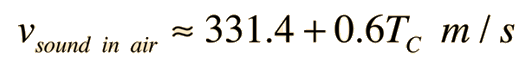# Longitudinal Waves - Kundt's Tube

Sound in air is propagated only by means of longitudinal waves; waves in which the particle's motion consists of oscillations back and forth in the direction of propagation. In a solid such as a metal rod, sound can be transmitted either by longitudinal or transverse waves. In this experiment, longitudinal sound waves will be produced in a metal rod and an air column. Using the properties of wave motion, the frequency of the sound and the speed of sound in the rod can be determined.The apparatus consists of a glass tube supported on a metal base as shown in the diagram. A clamp at one end of the base holds a metal rod which has a metal disk attached to one end. The rod and disk extend inside the glass tube, whose position can be adjusted to center the tube about the disk. It is important to make sure the disk is not touching the glass becuause the vibrations set up in the rod will break the glass. A stopper closes the other end of the tube. The velocity of any wave is given by:

v = fλ     (1)

where f is the frequency and λ is the wavelength. When the rod is properly stroked and set into vibration, standing waves are set up in the vibrating rod. Since the rod is clamped at its center point, this point is a node (zero amplitude of motion) and the ends which are free to vibrate are antinodes (maximum amplitude of particles' motion along the direction of the rod).

When the rod is vibrating in this manner it is vibrating with its fundamental frequency and the wavelength of the standing wave is twice the length of the rod.

The vibrations of the rod are transmitted by the disk to the air in the glass tube closed at one end.The waves set up in the air in the glass tube have the same frequency as those in the rod. The waves are reflected at the closed end of the tube and the air in the tube is thus acted upon by two similar sets of waves travelling in opposite directions. When the length of the air column is some multiple of half wavelengths, the two oppositely travelling waves produce standing waves. The standing waves are characterized by alternate points of maximum and minimum disturbance called respectively nodes and antinodes. These nodes and antinodes may be detected by cork dust placed in the tube, the cork dust showing characteristic striated vibration patterns at the antinodes. The distance between two successive patterns is therefore one-half the wave length of the sound in the air.

The wave length of the sound in air is thus determined. From tables the velocity of sound in air at the temperature of the room is obtained and using equation (1) the frequency may be computed. Since this is the frequency of the sound wave in the metal rod and since the wave length is twice the length of the rod, we can compute the velocity of the sound waves in the metal rod using equation (1).

Procedure: Place a thin layer of very dry cork dust at least two millimeters wide as uniformly as possible inside the tube along its entire length. It is necessary that the cork dust and the glass tube be perfectly dry. Insert the stopper in the end of the tube. With the rod securely clamped at its center, position the glass tube so that the disk on the end of the rod is a few centimeters within the tube and centered with respect to the sides.

Place a small amount of the rosin on a piece of leather cloth or chamois skin. Gripping the rod with the rosined leather, pull toward the end of the rod. Try to avoid pulling the cloth completely off the rod because this sets up transverse waves in the rod and may break the glass tube. Proper stroking sets the the rod into longitudinal vibrations and a note of high pitch will be heard. This stroking of the rod usually requires a little practice to produce satisfactory vibration, but is relatively easy once the proper technique is acquired. Slide the glass tube a short distance one way or the other while continuing the stroking until the most distinct dust heaps are obtained.

The distance between successive dust heaps is difficult to measure accurately because the exact center of the dust heap cannot be ascertained definitely. However, a good average can be obtained by measuring the distance over a large a number of dust heaps as possible and dividing by this number. Twice this value is the wave length of the sound. Record the room temperature and calculate the velocity of sound in air at this temperature:

 (2)Compute the frequency of the sound. Measure the length of the metal rod and using the measured frequency of the sound compute the velocity of sound in the metal rod. Compare this with handbook values for velocity of sound in this material.

A. Determination of sound frequency

 Data Brassrod Aluminumrod Steelrod 1. Distance between centers of cork dust patterns _______ _______ _______ 2. Wavelength in air _______ _______ _______ 3. Room temperature _______ _______ _______ 4. Velocity of sound in air at this temperature _______ _______ _______ 5. Frequency of sound _______ _______ _______

B. Determination of speed of sound in rod

 1. Length of rod _______ _______ _______ 2. Wavelength of sound in rod _______ _______ _______ 3. Speed of sound in rod _______ _______ _______ 4. Handbook value for speed of sound _______ _______ _______ 5. Measured speed in miles/hour. _______ _______ _______

## Equipment: Kundt's Tube

• Kundt's tube apparatus
• Two rods: aluminum, brass, or steel
• Piece of chamois
• Rosin
• Meter stickIndex

Apparatus movie
HyperPhysics***** Physics 2030K ***** Physics 2030K Laboratory Go Back